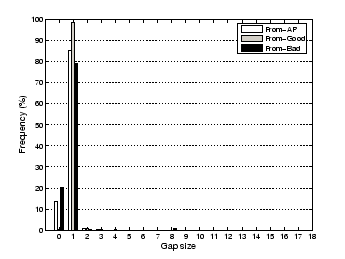Next: Conclusion Up: Analysis of WM Technique Previous: Application Layer Capture Accuracy

## MAC Layer Capture Accuracy

In this section, we examine WM's accuracy in measuring the MAC layer traffic. To count the measurement loss with only MAC frames, we exploited the IEEE 802.11 MAC sequence number. A wireless device increments the MAC sequence number whenever it sends a new Data/Management frame. We focus our analysis in this paper on the Data and Management frame types, as Control frames do not contain a sequence number. If a device retransmits a frame, then it uses the same sequence number as the original frame. Since the maximum MAC sequence number is 4095, a wireless device reuses the same sequence number every 4096 unique frames. We denote the difference in sequence numbers of two consecutive captured frames as the gap size. For example, if consecutive frames have sequence numbers, 4094 and 1, then there is a gap of size three () between the frames, and there are two missing frames between them.

 Adjusted From T U V T+U+V AP 97.85 96.88 96.14 98.53 98.5397.48 93.65 92.92 97.72 97.7261.09 88.51 88.67 88.96 93.31 Total 88.92 93.91 93.40 95.74 96.97

Table 2 analyzes the sequence numbers for the same controlled experiments as represented in Table 1. As MAC sequence numbers are generated per device, we examined the MAC sequence numbers of the AP, clientand clientseparately. Table 2 shows that as data from sniffers,, andare merged, the capture performance for AP,andincreases to 98.5%, 97.7%, and 89% respectively (with a further increase to 93% in the discussion below). The numbers in the table reflect the gap between different frames captured by the sniffers.Figure 3 shows histograms of the gap size between two consecutive frames for the case of using the three sniffers. Note that a gap of zero means that a frame has been retransmitted, while a gap of 1 means that there are no missing frames between these two frames. To calculate the number of missing frames, we count the gaps of greater than 1. Letdenote the number of occurrences of gap sizein Figure 3. Then, we can calculate the number of missing frames () by. The column labeled `T+U+V' in Table 2 can be obtained by, wheredenotes the number of distinct captured frames.Figure 4 takes a closer look at the Bad client case. The x-axis represents the received frame sequence number and the y-axis represents the gap before this frame. There was a periodic gap of 8, i.e., a measurement loss of 7 frames. By looking into the traces we found that these periodic behavior was due to the Bad client performing periodic active scanning searching for better APs. Since this process involves sending probe request frames on different channels 13 (13), the sequence numbers were not captured by our sniffers, which sniffed the traffic on only one channel. If these missed probe request frames are added to the loss statistics in Table 2, the capture accuracy for the Bad client increases to 93.31% and the overall capture performance increases to 96.97% (as shown in the Adjusted column in Table 2). This means that the WM statistics differ by at most 3.03% from the actual statistics.Next: Conclusion Up: Analysis of WM Technique Previous: Application Layer Capture Accuracy
Jihwang Yeo 2005-05-10